# Violin Plot for Data Analysis

Violin Plot is a method to visualize the distribution of numerical data of different variables. It is similar to Box Plot but with a rotated plot on each side, giving more information about the density estimate on the y-axis.
The density is mirrored and flipped over and the resulting shape is filled in, creating an image resembling a violin. The advantage of a violin plot is that it can show nuances in the distribution that aren’t perceptible in a boxplot. On the other hand, the boxplot more clearly shows the outliers in the data.

Violin Plots hold more information than the box plots, they are less popular. Because of their unpopularity, their meaning can be harder to grasp for many readers not familiar with the violin plot representation.

To get the link to Iris Data, click – here.

```Attribute Information:
-> sepal length in cm
-> sepal width in cm
-> petal length in cm
-> petal width in cm
-> class:
Iris Setosa
Iris Versicolour
Iris Virginica

Number of Instances: 150

Summary Statistics:
Min  Max   Mean    SD   Class Correlation
sepal length: 4.3  7.9   5.84  0.83    0.7826
sepal width: 2.0  4.4   3.05  0.43   -0.4194
petal length: 1.0  6.9   3.76  1.76    0.9490  (high!)
petal width: 0.1  2.5   1.20  0.76    0.9565  (high!)

Class Distribution: 33.3% for each of 3 classes.
```

 `import` `numpy as np ` `import` `pandas as pd ` `import` `seaborn as sns ` `from` `matplotlib ``import` `pyplot ` `import` `seaborn `

 `data ``=` `pd.read_csv(``"Iris.csv"``) ` ` `  `print` `(data.head(``10``)) `

Output: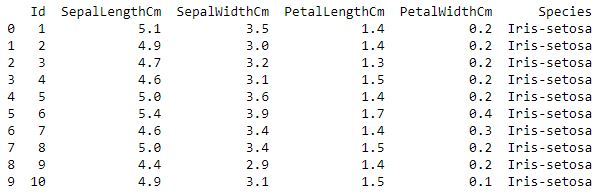Description

 `data.describe() `

Output: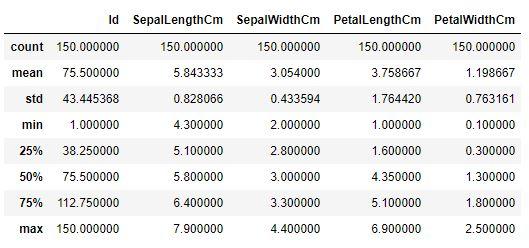Info

 `data.info() `

Output: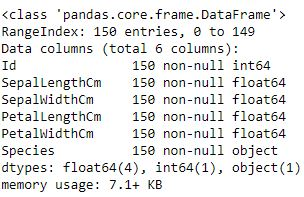Describing ‘SepalLengthCm’ parameter of Iris dataset.

 `data[``"SepalLengthCm"``].describe() `

Output:

```count    150.000000
mean       5.843333
std        0.828066
min        4.300000
25%        5.100000
50%        5.800000
75%        6.400000
max        7.900000
Name: SepalLengthCm, dtype: float64```

Code #1: Violin Plot for ‘SepalLengthCm’ Parameter.

 `fig, ax ``=` `pyplot.subplots(figsize ``=``(``9``, ``7``)) ` `sns.violinplot( ax ``=` `ax, y ``=` `data[``"SepalLengthCm"``] ) `

Output: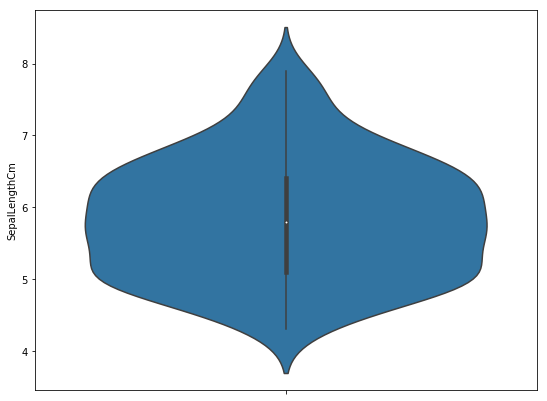As u can see we have a higher density between 5 and 6. That is very significant because as in the SepalLengthCm description, a mean value is at 5.43.

Code #2: Violin Plot for ‘SepalLengthWidth’ Parameter.

 `fig, ax ``=` `pyplot.subplots(figsize ``=``(``9``, ``7``)) ` `sns.violinplot(ax ``=` `ax,  y ``=` `data[``"SepalWidthCm"``] ) `

Output: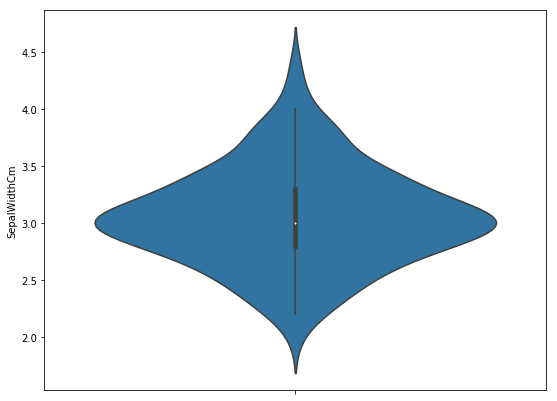Here also, Higher density is at the mean = 3.05

Code #3: Violin Plot comparing ‘SepalLengthCm’ and ‘SepalWidthCm’.

 `fig, ax ``=` `pyplot.subplots(figsize ``=``(``9``, ``7``)) ` `sns.violinplot(ax ``=` `ax, data ``=` `data.iloc[:, ``1``:``3``]) `

Output: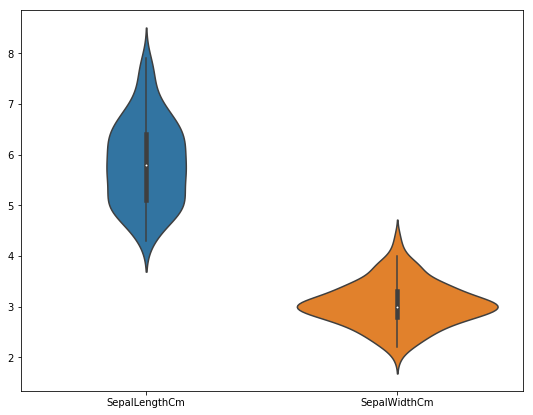Code #4: Violin Plot comparing ‘SepalLengthCm’ species wise.

 `fig, ax ``=` `pyplot.subplots(figsize ``=``(``9``, ``7``)) ` `sns.violinplot(ax ``=` `ax, x ``=` `data[``"Species"``],  ` `                  ``y ``=` `data[``"SepalLengthCm"``] ) `

Output: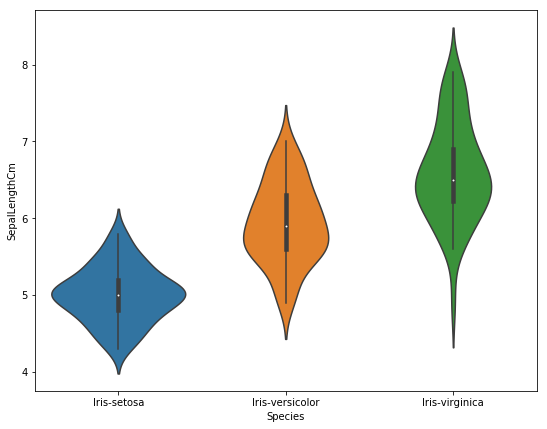My Personal Notes arrow_drop_upIf you like GeeksforGeeks and would like to contribute, you can also write an article using contribute.geeksforgeeks.org or mail your article to contribute@geeksforgeeks.org. See your article appearing on the GeeksforGeeks main page and help other Geeks.

Please Improve this article if you find anything incorrect by clicking on the "Improve Article" button below.

Improved By : ManasChhabra2

Article Tags :

Be the First to upvote.

Please write to us at contribute@geeksforgeeks.org to report any issue with the above content.